Courses

# NCERT Exemplar - Circles Class 10 Notes | EduRev

## Class 10 : NCERT Exemplar - Circles Class 10 Notes | EduRev

The document NCERT Exemplar - Circles Class 10 Notes | EduRev is a part of the Class 10 Course Mathematics (Maths) Class 10.
All you need of Class 10 at this link: Class 10

Q.1. Prove that the tangents drawn at the ends of a chord of circle make equal angles with the chord.
Ans.
Given: A circle with centre O, PA and PB are tangents drawn at the ends A and B on chord AB.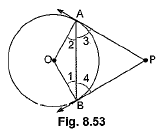To prove: ∠PAB = ∠PBA
Construction: Join OA and OB.
Proof: In ΔOAB we have
OA = OB ...(i) (Radii of the same circle)
∠2 = ∠1 ...(ii) (Angles opposite to equal sides of a Δ)
Also (∠2 + ∠3 = ∠1 + ∠4) ...(iii) (Both 90° as Radius ⊥ Tangent)
Subtracting (ii) from (iii), we have
∴ ∠3 = ∠4 ⇒ ∠PAB = ∠PBA

Q.2. The tangent at a point C of a circle and a diameter AB when extended intersect at P. If ∠PCA = 110°, find ∠CBA.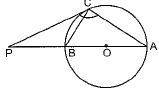Ans.
∠PCA =110°
∠CBA = ?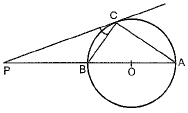∠ACB = 90° [Angle in a semicircle is right angle]
∠PCB = 110°- 90° = 20°
∠PCB = ∠CAB = 20°
[Alternate segment theorem]
In ΔABC,
∠ABC + ∠CAB + ∠BCA = 180°
[∵ Sum of angles of a Δ is 180°.]
⇒ ∠ABC = 180° - 110° = 70°.

Q.3. In figure, AB and CD are common tangents to two circles of unequal radii. Prove that AB = CD.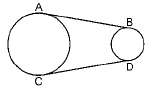Ans.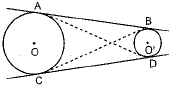Proof: The tangents drawn from an internal point to a circle are equal in length.
If A is external point for circle having center O'
If C is external point then
BC = CD ...(ii)
Now, B is external point for circle having center O
AB = BC ...(iii)
So, from (i), (ii) and (iii), we get
AB = BC = CD
So, AB = CD Hence proved.

Q.4.  From an external point P, two tangents, PA and PB are drawn to a circle with centre O. At one point E on the circle tangent is drawn which intersects PA and PB at C and D, respectively. If PA = 10 cm, find the perimeter of the triangle PCD.
Ans.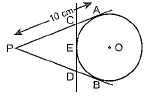PA = 10 cm.
PA = PB [If P is external point] ... (i)
[∵ From an external point tangents drawn to a circle are equal in length]
If C is external point, then CA = CE
If D is external point, then
DB = DE ...(ii)
Perimeter of triangle ΔPCD
= PC + CD + PD
= PC + CE + ED + PD
= PC + CA + DB + PD
= PA + PB
= PA + PA = 2 PA
= 2 x 10 = 20 cm. [From (i)]

Q.5. If AB is a chord of a circle with centre O, AOC is a diameter and AT is the tangent at A as shown in figure. Prove that ∠BAT = ∠ACB.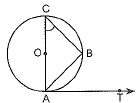Ans.
In ΔACB,
AC is diameter, ∠B = 90°
[In a semicircle there is always a right angle]
So, ∠ACB + ∠CAB = 90° ...(i)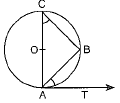[∵ sum of angles of a Δ is 180°]
OA ⊥ AT [Radius and tangent are ⊥ to each other at the point of contact]
∠OAT = 90°
∠OAB + ∠BAT = 90° ...(ii)
From (i) and (ii),
∠ACB = ∠BAT

Offer running on EduRev: Apply code STAYHOME200 to get INR 200 off on our premium plan EduRev Infinity!

## Mathematics (Maths) Class 10

50 videos|347 docs|138 tests

,

,

,

,

,

,

,

,

,

,

,

,

,

,

,

,

,

,

,

,

,

;